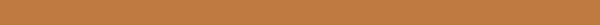# 现代量子力学笔记(三): 抽象到具体---真实的量子世界

## 测量

Dirac 1985, p.36

"A measurement always causes the system to jump into
an eigenstate of the dynamical variable that is being
measured."


## 对易非对易

$[A,B]=0,$则称算符A,B对易,$[A,B]\neq 0$称算符A,B非对易.

### 对易(Compatible)

A,B对易,则矩阵元$⟨a''|B|a'⟩$为对角矩阵.: $$⟨a''|B|a'⟩=δ_{a'a''}⟨a'|B|a'⟩.$$ 算符B写为: $$B=\sum_{a''}|a''⟩⟨a''|B|a''⟩⟨a''|.$$ B算符作用在A的其中一个本征向量$|a'⟩$上: $$B|a'⟩=\sum_{a''}|a''⟩⟨a''|B|a''⟩⟨a''|a'⟩=(⟨a'|B|a'⟩)|a'⟩$$ 说明A,和B有着相同的本征向量$|a'⟩$, $$b'=⟨a'|B|a'⟩$$

A,B的测量不会互相破坏信息(do not interfere),如下: $$|α⟩\xrightarrow{\text{A measure}}|a',b'⟩\xrightarrow{\text{B measure}}|a',b'⟩\xrightarrow{\text{A measure}}|a',b'⟩.$$

## 测不准关系(The Uncertainty Relation)